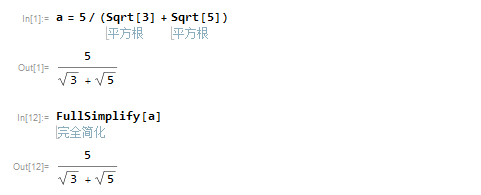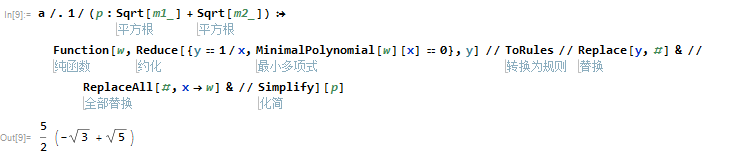# Mathematica分母有理化

• A+

``````a = 5/(Sqrt + Sqrt)
FullSimplify[a]````````````a /. 1/(p : Sqrt[m1_] + Sqrt[m2_]) :>
Function[w,
Reduce[{y == 1/x, MinimalPolynomial[w][x] == 0}, y] // ToRules //
Replace[y, #] & // ReplaceAll[#, x -> w] & // Simplify]``````• 微信公众号
• 关注微信公众号
•• QQ群
• 我们的QQ群号
•## 目前评论：1   其中：访客  1   博主  0

•累也快乐着

其实还可以使用MinimalPolynomial最小多项式命令来操作 更加简单